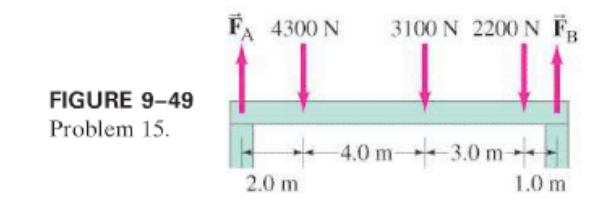×
Get Full Access to Physics: Principles With Applications - 6 Edition - Chapter 9 - Problem 15p
Get Full Access to Physics: Principles With Applications - 6 Edition - Chapter 9 - Problem 15p

×

# Calculate FA and FB for the beam shown in Fig. 9–49. TheISBN: 9780130606204 3

## Solution for problem 15P Chapter 9

Physics: Principles with Applications | 6th Edition

• Textbook Solutions
• 2901 Step-by-step solutions solved by professors and subject experts
• Get 24/7 help from StudySoup virtual teaching assistantsPhysics: Principles with Applications | 6th Edition

4 5 1 432 Reviews
22
3
Problem 15P

Calculate $$F_{A} \text { and } F_{B}$$ for the beam shown in Fig. 9–49. The downward forces represent the weights of machinery on the beam. Assume the beam is uniform and has a mass of 250 kg.Equation Transcription:Text Transcription:

F_A and F_B

Step-by-Step Solution:

SOLUTION:Step 1 of 3The momentum is quantity of measurement of motion of object.Mathematically, p=mv In an isolated system , the total momentum is conserved because there is no external force acting on it . Consider two bodies Initial momentum of the bodies before collision p and p respec1ively 2 final momentum of the bodies after collision p and p respec1vely 2 From newton's second law F= dp dt Force exerted on 1 by 2 ,F = p1p1 12 t Force exerted on 2 by 1 ,F = p2p2 21 t By newton's third law F 12=-F 21 p1p1 (p2p2) t = t p 1 p =1(p - p )2 2 p 1 p =p1+ p 2 2 p - p =p + p 1 2 1 2 p = p f i\n

Step 2 of 3

Step 3 of 3

##### ISBN: 9780130606204

This textbook survival guide was created for the textbook: Physics: Principles with Applications, edition: 6. The answer to “?Calculate $$F_{A} \text { and } F_{B}$$ for the beam shown in Fig. 9–49. The downward forces represent the weights of machinery on the beam. Assume the beam is uniform and has a mass of 250 kg. Equation Transcription:Text Transcription:F_A and F_B” is broken down into a number of easy to follow steps, and 42 words. The full step-by-step solution to problem: 15P from chapter: 9 was answered by , our top Physics solution expert on 03/03/17, 03:53PM. Since the solution to 15P from 9 chapter was answered, more than 785 students have viewed the full step-by-step answer. This full solution covers the following key subjects: beam, forces, calculate, downward, fig. This expansive textbook survival guide covers 35 chapters, and 3914 solutions. Physics: Principles with Applications was written by and is associated to the ISBN: 9780130606204.

## Discover and learn what students are asking

Calculus: Early Transcendental Functions : Basic Differentiation Rules and Rates of Change
?Finding a Derivative In Exercises 3–24, use the rules of differentiation to find the derivative of the function. $$y=12$$

Calculus: Early Transcendental Functions : Introduction to Functions of Several Variables
?In Exercises 19 - 30, find the domain and range of the function. $$f(x, y)=x^{2}+y^{2}$$

Unlock Textbook Solution